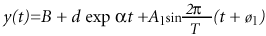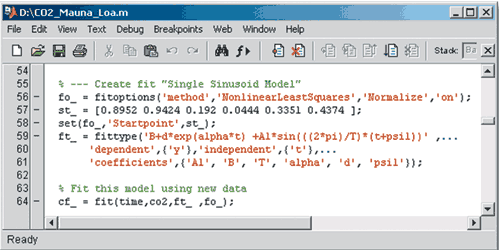# Atmospheric Carbon Dioxide Modeling and the Curve Fitting Toolbox

By Mary Ann Branch, MathWorks and Russ Minkwitz, MathWorks

A common measurement for studying the effect of burning fossil fuels on weather patterns is the level of carbon dioxide (CO2) concentration in the atmosphere. Some scientists believe the upward trend in CO2 levels could cause atmospheric temperatures to rise, polar ice caps to melt, and climates of different regions of the earth to change radically.

Since this data has cyclical phenomena, as well as an upward growth trend, nonlinear fitting techniques are appropriate, but can require added effort when compared to fitting a linear model. However, with the right tools and knowledge of your data, you can quickly determine the coefficients of a nonlinear model.

This article describes how to fit a nonlinear model to available CO2 data using the newly released Curve Fitting Toolbox.

### Modeling the Data

In this example, we use CO2 data, in parts per million, collected by the U.S. National Oceanic and Atmospheric Administration (NOAA) from 1979 to 1996 at the Mauna Loa Observatory in Hawaii2. The captured data is shown in the following illustration.The Curve Fitting Tool found in the Curve Fitting Toolbox gives an immediate visual display of the data. By using the Data Tips feature we can interrogate specific points.

Cyclical phenomena in nature can be modeled by a sinusoid and its higher harmonics, that is,AkT/K and Φk are the amplitude, period, and phase, respectively, of the k-th harmonic. The sinusoidal terms capture seasonal trends, but the persistent upward trend requires an exponential term. A suggested model for this data, determined by examining records of total world fossil fuel production from the mid 1860s, iswhere t (time) is in years, α is believed to be approximately 0.0444/year, and the other parameters are unknown (including the number of sinusoids needed)2.

To build the model, we go to the Curve Fitting Toolbox's Fitting GUI and create a custom equation with one sinusoid term.Because the time values are large, we can select the option to center and scale the time variable (remove the mean and divide by the standard deviation) to improve the conditioning of the numerical calculations.With the Custom Equation panel, we enter start values of α = .0444, T = 0.192 (the period of one year after centering and scaling), and random guesses for the rest of the parameters (below). When the fit completes and the result is plotted we see that the cyclical pattern has not been matched (left)The results displayed indicate that the R-square value (a measure of goodness-of-fit) is not too far from one, implying the global trend is met, but the local trend is not. In this case, the amplitude of the sinusoid term is too small.

### Refining the Model

We can improve upon this model in several ways. First, we can determine the coefficients of the exponential term separately. We use the toolbox's built-in exponential model which automatically calculates optimal start points from the data,Fitting this model results in coefficients of d = 349.6 and α = 0.02185. Using these values of d and a as start values in the sinusoidal model, we get a new fit that matches the cyclical nature well.The amplitude of this latest model is approximately three. We can verify on the plot that this is a reasonable value for A 1 (left). The Results panel (below) also shows the confidence intervals for each parameter in parentheses after the coefficient value. Smaller confidence intervals imply more confidence in the parameter values. The confidence intervals are small for all the parameters except for B and d, this may indicate that B and d are highly correlated. Now we can plot the residuals that still show a cyclical sinusoid pattern, so another sinusoid term is warranted.

Now we can plot the residuals that still show a cyclical sinusoid pattern, so another sinusoid term is warranted.Residuals of the single sinusoid model reveal yet another sinusoid term (left). With two harmonics, the fit is quite good (right). We can easily create equations with even more terms, examining the residuals of each resulting fit until we are satisfied.

### Extending the Analysis

When we have found a satisfactory model, we can send our fits to the MATLAB workspace for further analysis. We have several other observatory sites to examine (e.g., Samoa, the South Pole, and Barrow) and so can generate an M-file function that can recreate our fits. Then we can call the function in a loop to automatically fit other data sets to see how the resulting models compare across observatory sites.

This example touches on a few of the features that will help you fit models to data. Whether the models are linear or nonlinear, the Curve Fitting Toolbox enables you to quickly fit standard models from its library or custom models you create.M-file code to replicate the fits and plots created in the GUI can be generated from the GUI File menu. The M-file also reveals the toolbox commands that can be used at the command line to get the same results.

Published 2002 - 91034v00

### References

1. GLOBALVIEW-CO2, "Cooperative Atmospheric Data Integration Project - Carbon Dioxide" (2001). [Available to the public at ftp.cmdl.noaa.gov, Path: ccg/co2/GLOBALVIEW]

2. Kahner, D., Moler, C., Nash, S., Numerical Methods and Software, Prentice Hall (1989)